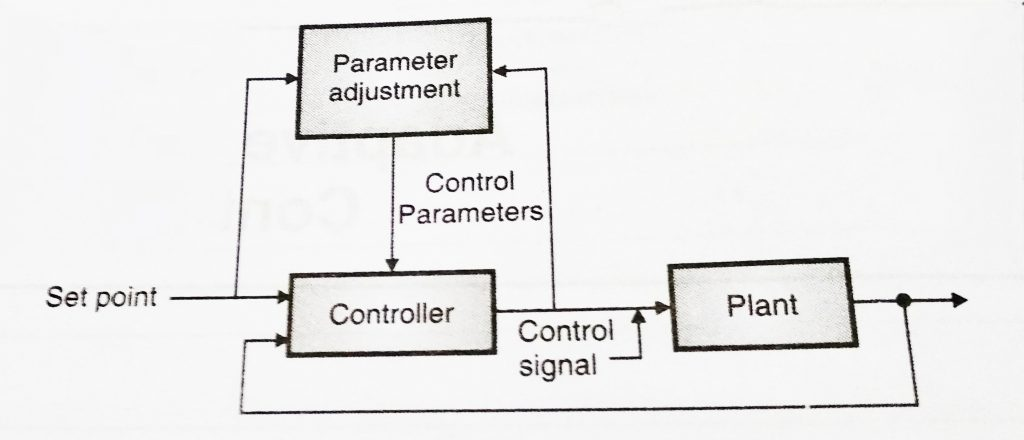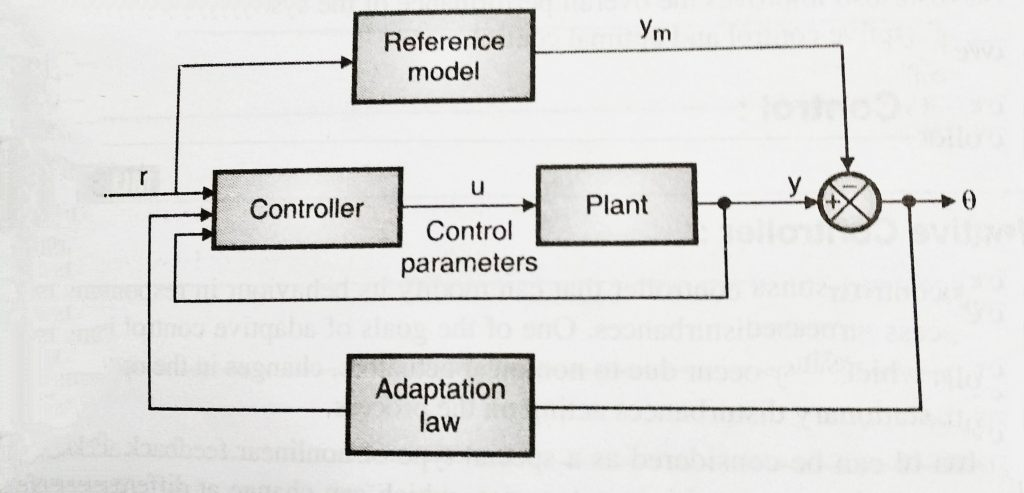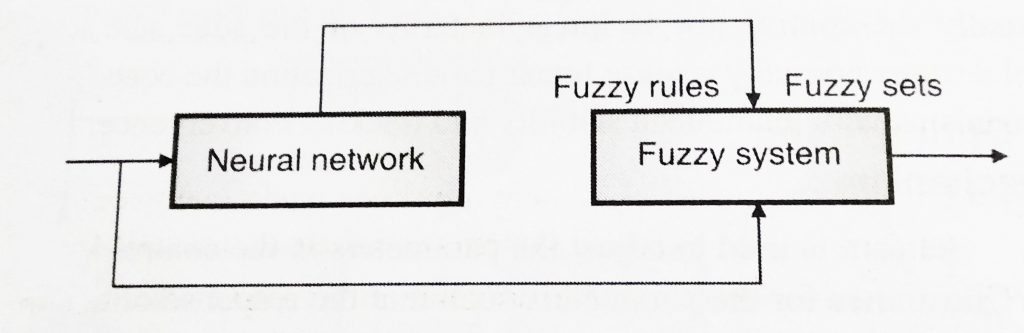# Control System – Adaptive and Optimal Control Systems

Advancement in today’s world is indeed required in every field, so is the case in control systems engineering. A process of continual review and improvement of how to take decision, allow the system to respond better manner to parameter variation and disturbances. It improved the overall performance of the system .

This controller can modify its behavior in response to changes in the dynamics of the process and the disturbances. Its main goal is to compensate for the parameter variation(which may occur due to nonlinear actuators or the operating conditions).

It is further considered as the special type of nonlinear feedback control in which the multiple stages of any process can be separated into different categories, which can change at any different rates.

An adaptive controller is a type of controller along with the adjustable parameters and also a mechanism in order to adjust the respective parameters. It can be considered as consist of two loops, one loop is normal feedback with the process and controller.The other loop is a parameter adjustment loop you can refer the below diagram for better clarity:This is an adaptive servo system in which the desired performance is expressed in terms of the reference model, which gives desired response to the reference signal. You can refer below diagram:MARC basically consists of a plant containing unknown parameters , also a reference model for compactly specifying the desired output of the control system, a feedback control law containing the adjustable parameters . The ordinary feedback loop is further known as the inner loop and the parameter adjustment loop is called the outer (external) loop.

A reference model is utilized to showcase the ideal response for the adaptive control system to the external command .the controller is usually parameterized by a number of adjustable parameters. Adaptive control design normally require  linear parameterization of the controller in order to obtain an adaptation mechanism. The design of MARC normally includes the following three steps:

•  First select a control law containing variable parameters
• Then choose an adaptation law in order to adjust the parameters.
• Analyse the convergence properties of the resulting closed loop control system.

As the name suggests, this type of control combines two paradigms i.e Fuzzy logic and Neural networks. They are often considered as part of soft computing.

The term Neuro Fuzzy is associated with hybrid systems that act on two distinct subproblems : a neural network is utilized in first subproblem and a fuzzy logic system is utilized in the second sub problem.there are several different implementations of neuro-fuzzy systems. One such simplified block diagram of a neuro-fuzzy controller is shown below:In the above technique, the neural network learning mechanism determines the fuzzy rules from the training set. In general the neuro-fuzzy  term means a type of system where fuzzy sets and rules are adjusted using neural networks tuning techniques in an iterative way with data vectors. If you are more interested in this area , ten you may refer some reference books or sites to gain in-depth knowledge in this area.